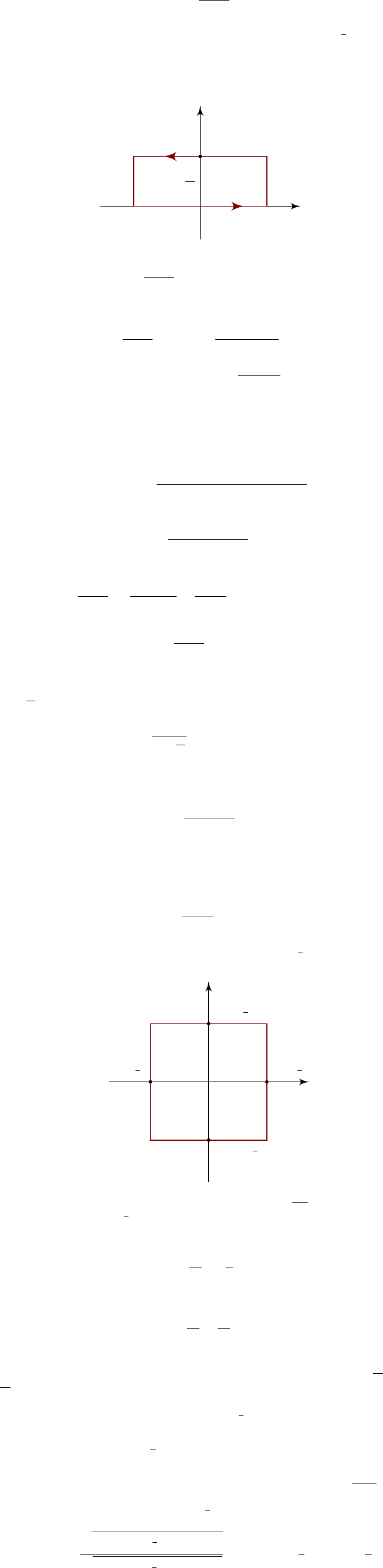4The calculus of residues

IB Complex Methods4.3 Further applications of the residue theorem using rect-
angular contours
Example. We want to calculate
I =
Z
−∞
e
αx
cosh x
dx,
where
α
(
1
,
1). We notice this has singularities at
x
=
n +
1
2
πi
for all
n
. So if we used our good, old semi-circular contours, then we would run into
infinitely many singularities as R .
Instead, we abuse the periodic nature of
cosh
, and consider the following
rectangular contour:
R
γ
0
R
γ
+
R
γ
1
γ
R
×
πi
2
πi
We see that
Z
γ
0
e
αz
cosh z
dz I as R .
Also,
Z
γ
1
e
αz
cosh z
dz =
Z
R
R
e
α(x+πi)
cosh(x + πi)
dx
= e
απi
Z
R
R
e
αx
cosh x
dx
e
απi
I.
On the γ
+
R
, we have
cosh z = cosh(R + iy) = cosh R cos y + i sinh R sin y.
So
|cosh z| =
q
cosh
2
R cos
2
y + sinh
2
R sin
2
y.
We now use the formula cosh
2
R = 1 + sinh
2
R, and magically obtain
|cosh z| =
q
cos
2
y + sinh
2
R sinh R.
Hence
e
αz
cosh z
|e
αR
e
αiy
|
sinh R
=
e
αR
sinh R
= O(e
(α1)R
) 0.
Hence as R ,
Z
γ
+
e
αz
cosh z
dz 0.
Similarly, the integral along γ
vanishes.
Finally, we need to find the residue. The only singularity inside the contour
is at
πi
2
, where the residue is
e
απi/2
sinh
πi
2
= ie
απi/2
.
Hence we get
I(1 + e
απi
) = 2πi(ie
απi/2
) = 2πie
απi/2
.
So we find
I =
π
cos(απ/2)
.
These rectangular contours tend to be useful for trigonometric and hyperbolic
functions.
Example. Consider the integral
Z
γ
cot πz
z
2
dz,
where
γ
is the square contour shown with corners at (
N
+
1
2
)(
±
1
± i
), where
N
is a large integer, avoiding the singularities
× × × × × × × × × × ×
(N +
1
2
)i
(N +
1
2
)i
N +
1
2
(N +
1
2
)
There are simple poles at
z
=
n Z \ {
0
}
, with residues
1
n
2
π
, and a triple pole
at
z
= 0 with residue
1
3
π
(from the Taylor series for
z
2
tan πz
). It turns out
the integrals along the sides all vanish as N (see later). So we know
2πi
2
N
X
n=1
1
n
2
π
π
3
!
0
as N . In other words,
N
X
n=1
1
n
2
=
π
2
6
.
This is probably the most complicated and most inefficient way of computing
this series. However, notice we can easily modify this to fund the sum of
1
n
3
, or
1
n
4
, or any complicated sum we can think of.
Hence all that remains is to show that the integrals along the sides vanish.
On the right-hand side, we can write z = N +
1
2
+ iy. Then
|cot πz| =
cot

N +
1
2
+ y
= | tan y| = |tanh πy| 1.
So
cot πz
is bounded on the vertical side. Since we are integrating
cot πz
z
2
, the
integral vanishes as N .
Along the top, we get z = x +
N +
1
2
i. This gives
|cot πz| =
q
cosh
2
N +
1
2
π sin
2
πx
q
sinh
2
N +
1
2
π + sin
2
πx
coth
N +
1
2
π coth
π
2
.
So again
cot πz
is bounded on the top side. So again, the integral vanishes as
N .